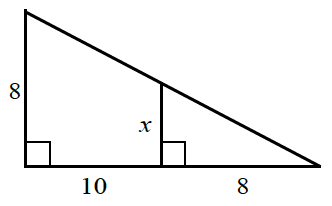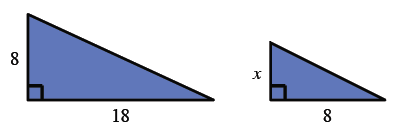### Home > CC2MN > Chapter 11 > Lesson 11.1.5 > Problem11-69

11-69.

Examine the diagram below. The smaller triangle is similar to the larger triangle. Write and solve a proportion to find $x$. It may be helpful to draw the two triangles separately.Draw two separate triangles. The larger triangle has base $10+8=18$.$\frac{x}{8}=\frac{8}{18}$